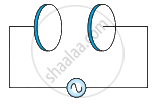# A parallel plate capacitor (Figure) made of circular plates each of radius R = 6.0 cm has a capacitance C = 100 pF. The capacitor is connected to a 230 V - Physics

Numerical

A parallel plate capacitor (Figure) made of circular plates each of radius R = 6.0 cm has a capacitance C = 100 pF. The capacitor is connected to a 230 V ac supply with a (angular) frequency of 300 rad s−1.

(a) What is the rms value of the conduction current?

(b) Is the conduction current equal to the displacement current?

(c) Determine the amplitude of B at a point 3.0 cm from the axis between the plates.#### Solution

Radius of each circular plate, R = 6.0 cm = 0.06 m

Capacitance of a parallel plate capacitor, C = 100 pF = 100 × 10−12 F

Supply voltage, V = 230 V

Angular frequency, ω = 300 rad s−1

(a) Rms value of conduction current, I = "V"/"X"_"C"

Where,

XC = Capacitive reactance

= 1/(ω"C")

∴ I = V × ωC

= 230 × 300 × 100 × 10−12

= 6.9 × 10−6 A

= 6.9 μA

Hence, the rms value of conduction current is 6.9 μA.

(b) Yes, conduction current is equal to displacement current.

(c) Magnetic field is given as:

B = (μ_0"r")/(2pi"R"^2)"I"_0

Where,

μ0 = Free space permeability = 4π × 10−7 N A−2

I0 = Maximum value of current = sqrt2"I"

r = Distance between the plates from the axis = 3.0 cm = 0.03 m

∴ B = (4pi xx 10^-7 xx 0.03 xx sqrt2 xx 6.9 xx 10^-6)/(2pi xx (0.06)^2)

= 1.63 × 10−11 T

Hence, the magnetic field at that point is 1.63 × 10−11 T.

Concept: Displacement Current
Is there an error in this question or solution?

#### APPEARS IN

NCERT Physics Part 1 and 2 Class 12
Chapter 8 Electromagnetic Waves
Exercise | Q 8.2 | Page 286
NCERT Class 12 Physics Textbook
Chapter 8 Electromagnetic Waves
Exercise | Q 2 | Page 286

Share